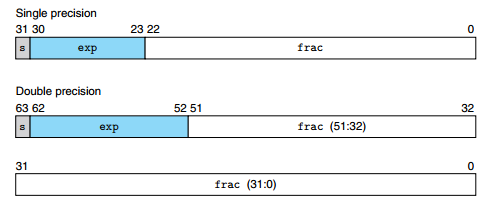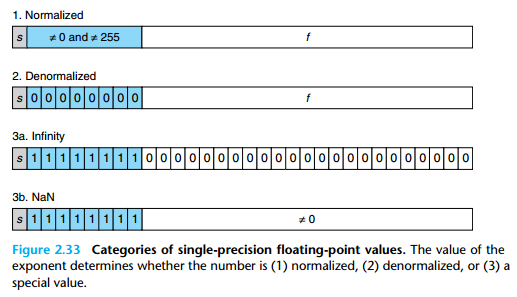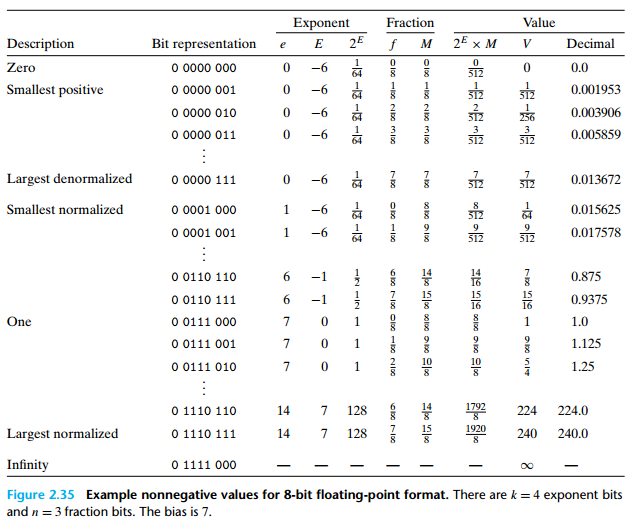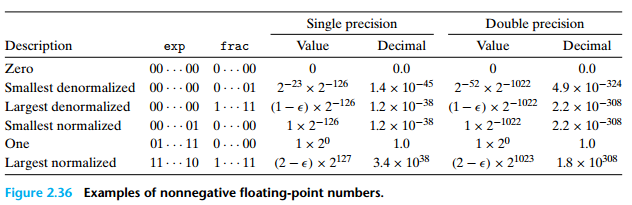# 浮点数

## 1. IEEE 754 标准

IEEE 754 浮点标准用 $V = (-1)^s \times M \times 2^E$ 的形式来表示一个数。• 符号位（s）：指明数的正负，即 $V$ 中的 $s$

• 阶码（exp）：对浮点数加权，即 $V$ 中的 $E$

• 尾数（frac）：二进制小数，即 $V$ 中的 $M$，范围为 $1 \sim 2 - \varepsilon$（规格化数），或者是 $0 \sim 1 - \varepsilon$（非规格化数），其中 $\varepsilon = 2^{-|frac|}$$|frac|$ 为尾数所占有的位数。

## 2. 浮点数数值分类

• 以单精度为例：### 2.1 规格化的值

• 阶码的值 $E = e - Bias$ 。其中 $e$ 为阶码的位模式对应的无符号数；$Bias = 2^{k-1} - 1$$k$ 为阶码的位数（单精度 $Bias = 127$、双精度 $Bias = 1023$）。

• 尾码的值 $M = 1 + f$$f$$frac = f_{n-1} \cdots f_1 f_0$ 描述的小数值 $0.f_{n-1} \cdots f_1 f_0$$n = |frac|$。即 $M = 1.f_{n-1} \cdots f_1 f_0$，在计算机里以 $frac$ 表示时去掉了总为 1 的首位（节省了一位空间）。

### 2.2 非规格化的值

• 阶码的值 $E = 1 - Bias$$Bias = 2^{k-1} - 1$$k$ 为阶码的位数（单精度 $Bias = 127$、双精度 $Bias = 1023$）。

• 尾码的值 $M = f$$f$$frac = f_{n-1} \cdots f_1 f_0$ 描述的小数值 $0.f_{n-1} \cdots f_1 f_0$

### 非规格化数的作用

#### 1. 提供了一种表示 0 的方法

• $+0.0$：符号位为 0，阶码为全 0，尾数为全 0 。
• $-0.0$：符号位为 1，阶码为全 0，尾数为全 0 。

【注】IEEE 的浮点格式中，$+0.0$$-0.0$ 在某些方面被认为是不同的，而在其他方面是相同的。

### 2.3 特殊值

• 尾数全为 0：得到的值表示无穷。当 $s = 0$ 是为 $+\infty$；当 $s = 1$ 是为 $-\infty$ 。用于表示溢出的结果，比如两个非常大的数相乘溢出了。

• 尾数为非 0：得到的值表示不是一个数值，即 $NaN$ 。用于表示一些非法的运算结果，如 $\sqrt{-1}$$\infty - \infty$ 等，也可以用来表示未初始化的值。

### 2.4 举例## 3. 浮点数数值舍入

IEEE 浮点格式定义了四种不同格式的舍入方式，默认的方法是向偶数舍入。

### 3.1 向上舍入

$\hat{x} = \lceil x \rceil$

### 3.2 向下舍入

$\hat{x} = \lfloor x \rfloor$

• x > 0：向下舍入。
• x < 0：向上舍入。

## 4. 运算

### 4.1 浮点加法

• 满足交换律：$x +^f y = y +^f x$
• 基本满足单调性：$\forall x \ne NaN, a \geq b \iff a + x \geq b + x$
• 存在单位元：$0.0$
• 基本存在逆元：$x +^f (-x) = 0.0$
除了 $NaN, \pm \infty$ 不满足有逆元外，其他数值都满足存在逆元。
• 不满足可结合性：由于存在精度损失，导致浮点数加法无法满足可结合性，比如：

$\begin{array}{c} (3.14 + 10^{10}) - 10^{10} = 0.0 \\ 3.14 + (10^{10} - 10^{10}) = 3.14 \end{array}$

### 4.2 浮点乘法

• 满足交换律：$x \times^f y = y \times^f x$
• 基本满足单调性：$\forall x \ne NaN, a \geq b \iff ((x \geq 0) \land (a \times^f x \geq b \times^f x)) \lor ((x \leq 0) \land (a \times^f x \leq b \times^f x))$
• 存在单位元：$1.0$
• 不满足可结合性：

$\begin{array}{c} (10^{20} \times 10^{20}) \times 10^{-20} = +\infty \\ 10^{20} \times (10^{20} \times 10^{-20}) = 10^{20} \end{array}$

• 对加法不满足分配律：

$\begin{array}{c} 10^{20} \times (10^{20} - 10^{20}) = 0.0 \\ 10^{20} \times 10^{20} - 10^{20} \times 10^{20} = NaN \end{array}$

### 4.3 精度

• 对于规格化数：单精度浮点数有效位数为 24 位，双精度浮点数有效位数为 53 位（隐含尾数未显示的首位 1 ）。

• 对于非规格化数：单精度浮点数有效位数为 23 位， 双精度浮点数有效位数为 52 位。

$\begin{array}{c} (1 \lt\lt 24) + 1 = (1 \lt\lt 24) \end{array}$